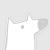Scala 是一门现代的多范式编程语言，志在以简练、优雅及类型安全的方式来表达常用编程模式。它平滑地集成了面向对象和函数语言的特性。Scala 运行于Java 平台（Java 虚拟机），并兼容现有的 Java 程序。

### 热门内容0+6+0+

### 最新内容0+0+0+

### 最新论文We consider the problem of training machine learning models over multi-relational data. The mainstream approach is to first construct the training dataset using a feature extraction query over input database and then use a statistical software package of choice to train the model. In this paper we introduce Iterative Functional Aggregate Queries (IFAQ), a framework that realizes an alternative approach. IFAQ treats the feature extraction query and the learning task as one program given in the IFAQ's domain-specific language, which captures a subset of Python commonly used in Jupyter notebooks for rapid prototyping of machine learning applications. The program is subject to several layers of IFAQ optimizations, such as algebraic transformations, loop transformations, schema specialization, data layout optimizations, and finally compilation into efficient low-level C++ code specialized for the given workload and data. We show that a Scala implementation of IFAQ can outperform mlpack, Scikit, and TensorFlow by several orders of magnitude for linear regression and regression tree models over several relational datasets.0+0+0+下载预览

Top